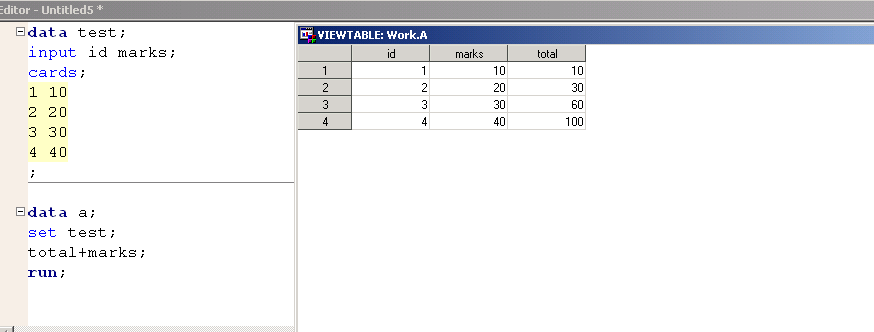## proc sql

Hi All,1 ACCEPTED SOLUTION

Accepted Solutions

## Re: proc sql

```data test;
input id marks;
cards;
1 10
2 20
3 30
4 40
;
run;
proc sql;
create table WANT as
select  *,
(select sum(MARKS) from TEST where ID <= A.ID) as TOTAL
from    TEST A;
quit;```
7 REPLIES 7

## Re: proc sql

Why do you need to use SQL?  Datastep is far better suited to this.  Untested code here, post test data in the form of a datastep as text in a code window ({i} above post)!!

```proc sql;
create table WANT as
select  *,
(select sum(MARKS) from TOTAL where ID <= A.ID) as TOTAL
from    TEST A;
quit;```

data test;
input id marks;
cards;
1 10
2 20
3 30
4 40
;

## Re: proc sql

```data test;
input id marks;
cards;
1 10
2 20
3 30
4 40
;
run;
proc sql;
create table WANT as
select  *,
(select sum(MARKS) from TEST where ID <= A.ID) as TOTAL
from    TEST A;
quit;```

## Re: proc sql

If you dont mind can you please explain this query, how (select sum(MARKS) from TEST where ID <= A.ID) as TOTAL
from TEST A;

is working. Please its a request ...

because i am unable to figure out how the id is comparing to a.id ..because we are not using the self join here

## Re: proc sql

Its called a subquery.  A.ID is from the outer query, the sub query is getting all records with ID less than the row from A and summing them up.

## Re: proc sql

i wrote the below query and its working fine but i have compared and the group by them..But in your query how it is getting grouped ...

proc sql;
select distinct test.marks,test.id ,sum(a.marks) as total from test,test as a where a.marks le test.marks group by test.id;
quit;

## Re: proc sql

It is not being "grouped" it is a sub-query.  So one first iteration, the subquery sums() up everything with an id < 10 and returns that, then next iteration id <20 returns that on and on.  You may want to have a readup on SQL and how it works.

Discussion stats
• 7 replies
• 966 views
• 1 like
• 2 in conversation